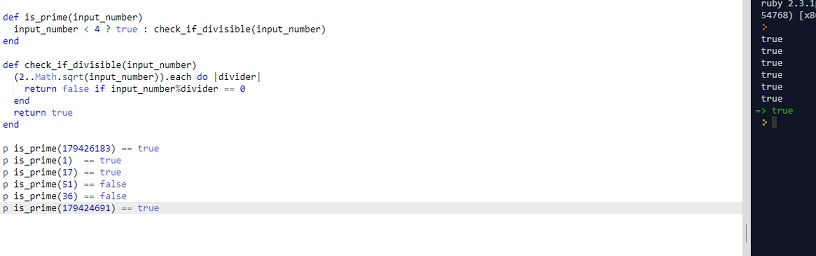# CLEAN CODE: CHECK IF A NUMBER IS PRIME

By: Saurav

2017-11-10 00:29:00 UTC

This is probably the first question interviewer asks as ice breaker. It has been asked to me as well in first telephonic interviews.

Lets tackle this problem and write a clean code for this one:

Lets write some tests first:

```p is_prime(179426183) == true
p is_prime(1)  == true
p is_prime(17) == true
p is_prime(51) == false
p is_prime(36) == false
p is_prime(179424691) == true```

Attempt 1:

```def is_prime(input_number)
return true if input_number < 4
return check_if_divisible(input_number)
end

def check_if_divisible(input_number)
(2..Math.sqrt(input_number)).each do |divider|
return false if input_number%divider == 0
end
return true
end ```

The main idea is to understand that we only need to check if a number is prime from 2 to its square root. If we go further down the number line after its square root on its right, we will meet the same quotient as divisor.

Why waste resource then!```def is_prime(input_number)
input_number < 4 ? true : check_if_divisible(input_number)
end

def check_if_divisible(input_number)
(2..Math.sqrt(input_number)).each do |divider|
return false if input_number%divider == 0
end
return true
end
```

Attempt 2 is just a refactored inline code as above.

So the function is essentially a one line code.

It turns out that 1 and 0 should not be considered prime.

No probs! Lets add one more line to the code and make few changes in the tests as well.

Attempt 3:

```def is_prime(input_number)
return false if input_number < 2
input_number < 4 ? true : check_if_divisible(input_number)
end

def check_if_divisible(input_number)
(2..Math.sqrt(input_number)).each do |divider|
return false if input_number%divider == 0
end
return true
end
```

```p is_prime(179426183) == true
p is_prime(1)  == false
p is_prime(0)  == false
p is_prime(17) == true
p is_prime(51) == false
p is_prime(36) == false
p is_prime(179424691) == true```

### Let me know what you think! twitter: sprakash24octlinkedin

Owned & Maintained by Saurav Prakash

If you like what you see, you can help me cover server costs or buy me a cup of coffee though donation :)